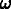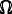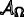# Blocking an Argument for Emergent Chance

## Abstract

Several authors have argued that non-extreme probabilities used in special sciences such as chemistry and biology can be objective chances, even if the true microphysical description of the world is deterministic. This article examines an influential version of this argument and shows that it depends on a particular methodology for defining the relationship between coarse-grained and fine-grained events. An alternative methodology for coarse-graining is proposed. This alternative methodology blocks this argument for the existence of emergent chances, and makes better sense of two well-known subjects of philosophical discussion: the Miners Puzzle and Simpson’s Paradox.

This is a preview of subscription content, access via your institution.

## References

1. 1.

Loewer, B. (2001). Determinism and chance. Studies in History and Philosophy of Science Part B, 32(4), 609–620.

2. 2.

Cohen, J., & Callender, C. (2009). A better best system account of lawhood. Philosophical Studies, 145(1), 1–34.

3. 3.

Hoefer, C. (2007). The third way on objective probability: A sceptic’s guide to objective chance. Mind, 116(463), 549–596.

4. 4.

Hoefer, C. (2019). Chance in the world: A humean guide to objective chance. Oxford: Oxford University Press.

5. 5.

Sober, E. (2010). Evolutionary theory and the reality of macro probabilities. In Eells, E, & Fetzer, JH (Eds.) The place of probability in science, (pp. 133–60). Springer.

6. 6.

Glynn, L. (2010). Deterministic chance. British Journal for the Philosophy of Science, 61(1), 51–80.

7. 7.

Frigg, R., & Hoefer, C. (2015). The best humean system for statistical mechanics. Erkenntnis, 80(3), 551–574.

8. 8.

List, C., & Pivato, M. (2015). Emergent chance. Philosophical Review, 124(1), 119–152.

9. 9.

Hemmo, M., & Shenker, O. (2019). Two kinds of high-level probability. The Monist, 102(4), 458–477.

10. 10.

Kolodny, N., & MacFarlane, J. (2010). Ifs and oughts. The Journal of philosophy, 107(3), 115–143.

11. 11.

List, C., & Pivato, M. (2021). Dynamic and stochastic systems as a framework for metaphysics and the philosophy of science. Synthese, 198, 2551–2612.

12. 12.

Gyenis, Z., & Rédei, M. (2017). General properties of bayesian learning as statistical inference determined by conditional expectations. The Review of Symbolic Logic, 10(4), 719–755.

13. 13.

Schaffer, J. (2007). Deterministic chance?. British Journal for the Philosophy of Science, 58(2), 113–140.

14. 14.

Kotzen, M. (2013). Conditional oughts and simpson’s paradox. Unpublished manuscript.

15. 15.

Simpson, E.H. (1951). The interpretation of interaction in contingency tables. Journal of the Royal Statistical Society. Series B (Methodological), 13 (2), 238–241.

16. 16.

Yule, G.U. (1903). Notes on the theory of association of attributes in statistics. Biometrika, 2(2), 121–134.

17. 17.

Cartwright, N. (1979). Causal laws and effective strategies. Nous, 13(4), 419–437.

18. 18.

Sprenger, J., & Weinberger, N. (2021). Simpson’s paradox. In Zalta, E.N. (Ed.) The stanford encyclopedia of philosophy. Metaphysics Research Lab, Stanford University, Summer 2021.

19. 19.

Chuang, J.S., Rivoire, O., & Leibler, S. (2009). Simpson’s paradox in a synthetic microbial system. Science, 323(5911), 272–275.

20. 20.

Sober, E., & Wilson, D.S. (1998). Unto others: The evolution and psychology of unselfish behavior. Cambridge: Harvard University Press.

21. 21.

Fitelson, B. (2017). Confirmation, causation, and simpson’s paradox. Episteme, 14(3), 297–309.

22. 22.

Shalizi, C.R., & Moore, C. (2003). What is a macrostate? subjective observations and objective dynamics. arXiv preprint cond-mat/0303625.

23. 23.

Lyon, A. (2011). Deterministic probability: Neither chance nor credence. Synthese, 182(3), 413–432.

24. 24.

Strevens, M. (2011). Probability out of determinism. In Beisbart, C, & Hartmann, S (Eds.) Probabilities in physics, (pp. 339–364). Oxford University Press.

25. 25.

Canson, C.D. (forthcoming). Objectivity and the method of arbitrary functions. The British Journal for the Philosophy of Science. The British Journal for the Philosophy of Science. https://www.journals.uchicago.edu/doi/abs/10.1093/bjps/axaa001.

26. 26.

Ismael, J.T. (2009). Probability in deterministic physics. Journal of Philosophy, 106(2), 89–108.

27. 27.

Ismael, J. (2011). A modest proposal about chance. The Journal of philosophy, 108(8), 416–442.

28. 28.

Hemmo, M., & Shenker, O.R. (2012). The road to maxwell’s demon: conceptual foundations of statistical mechanics. Cambridge: Cambridge University Press.

29. 29.

Shenker, O. (2017). Foundation of statistical mechanics: mechanics by itself. Philosophy Compass, 12(12), e12465.

30. 30.

List, C. (2019). Levels: descriptive, explanatory, and ontological. Noûs, 53(4), 852–883.

Download references

## Author information

Authors

### Corresponding author

Correspondence to David Kinney.

## Additional information

### Publisher’s Note

Springer Nature remains neutral with regard to jurisdictional claims in published maps and institutional affiliations.

I am grateful to Jonathan Birch, Luc Bovens, Chloé de Canson, Paul Daniell, Phil Dowe, Christopher Hitchcock, Tyler Millhouse, Jeremy Strasser, Katie Steele, David Watson, James Wills, two anonymous reviewers, and audiences at the 2017 meeting of the Australasian Association of Philosophy in Adelaide, the HPS Seminar at the University of Melbourne, and the LSE Choice Group for their feedback on various versions of this paper.

## Appendix

### Proof

Let ω ∈Ω be any possible world. Recall that ω is a mapping $$T\rightarrow S$$. Let a “conjoined pair” of states (si,sj) ∈ S be any pair of states such that, according to the coarsening function. Let $${\Omega }^{\prime }_{k}\subseteq {\Omega }$$ be a set of possible worlds such that, for any pair of worlds $$(\omega _{l},\omega _{m})\in {\Omega }^{\prime }_{k}$$ and all t, the states ωl(t) and ωm(t) are conjoined. The set of such sets of worlds $${\Omega }^{\dagger }=\{{\Omega }^{\prime }_{1},{\Omega }^{\prime }_{2},...,{\Omega }^{\prime }_{n}\}$$ fully partitions the set of all possible worlds Ω. If the coarsening function σ(⋅) is applied to the set of states S, then each set of fine-grained worlds $${\Omega }^{\prime }_{k}$$ is replaced by a single coarse-grained world. Thus, we can define a bijection from Ω into the set of coarse-grained possible worlds, and therefore a bijection from $$\mathcal {A}_{{\Omega }^{\dagger }}$$ into.

Finally, define a coarsening function $$\phi (\cdot ):\mathcal {A}_{\Omega }\rightarrow \mathcal {A}_{\Omega }$$ such that $$\mathcal {A}_{{\Omega }^{\dagger }}$$ is the range of ϕ(⋅), i.e. $${\Gamma }_{\phi (\cdot )}=\mathcal {A}_{{\Omega }^{\dagger }}$$. It follows that there is a bijection between Γϕ(⋅) and. For any $$E\in \mathcal {A}_{\Omega }$$ and any $$\phi (E)\in \mathcal {A}_{{\Omega }^{\dagger }}$$, if ωE, then ωϕ(E), since any ω is conjoined with itself. Thus, in keeping with my proposed restriction on any coarsening function ϕ(⋅), for any $$E\in \mathcal {A}_{\Omega }$$, $$E\subseteq \phi (E)$$. □

## Rights and permissions

Reprints and Permissions